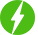# Understanding Calculus : A Graphical Approach

0

Understanding Calculus : A Graphical Approach, Visualizing Concepts of Calculus: Functions, Limits, Continuity, Differentiation, Integration, Differential Equations.

## Course Description

Calculus is the study of rates of change. Calculus was developed in the latter half of the 17th century by two mathematicians, Leibniz and Newton.

It was Newton who first developed calculus and applied it directly to the understanding of physical systems. Independently, Leibniz developed the notations used in calculus. In this Course we will Study

1. Real Valued Functions of Real Variables and Graphs of Standard Functions

2. Limits of Functions

3. Continuity of Functions

4. Differentiation of Functions

5. Mean Value Theorems

6. Riemann Integration

We will be happy to hear your thoughtsRegister New Account
• Total (0)
0